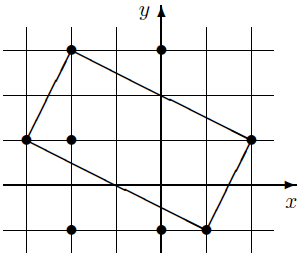## Day 2, Problem 1: Rectangle

You are given n points in the coordinate plane.

Write a program which calculates the largest possible area of a rectangle such that each of its vertices is one of the given points. You may assume that such a rectangle exists.

### Input

The input is read from standard input. The first line of input contains an integer n (4 ≤ n ≤ 1500), the number of given points.

Each of the following n lines contains the coordinates of one point, two integers separated by a space. The coordinates will be between -108 and 108.

No two points will be located at the same coordinates.

### Output

Output should be written to standard output. The first and only line of output should contain a single integer, the largest possible area of a rectangle.

```8
-2 3
-2 -1
0 3
0 -1
1 -1
2 1
-3 1
-2 1```

`10`

### IllustrationFor test cases worth 20% of the total score, n ≤ 500.

Point Value: 20 (partial)
Time Limit: 8.00s
Memory Limit: 64M